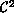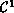Electron. J. Diff. Eqns., Monograph 02, 2000.

### Linearization via the Lie Derivative Carmen Chicone & Richard Swanson

Abstract:
The standard proof of the Grobman--Hartman linearization theorem for a flow at a hyperbolic rest point proceeds by first establishing the analogous result for hyperbolic fixed points of local diffeomorphisms. In this exposition we present a simple direct proof that avoids the discrete case altogether. We give new proofs for Hartman's smoothness results: Aflow islinearizable at a hyperbolic sink, and aflow in the plane islinearizable at a hyperbolic rest point. Also, we formulate and prove some new results on smooth linearization for special classes of quasi-linear vector fields where either the nonlinear part is restricted or additional conditions on the spectrum of the linear part (not related to resonance conditions) are imposed.

Submitted November 14, 2000. Published December 4, 2000.
Math Subject Classifications: 34-02, 34C20, 37D05, 37G10.
Key Words: Smooth linearization, Lie derivative, Hartman, Grobman, hyperbolic rest point, fiber contraction, Dorroh smoothing.

Show me the PDF file (426K), TEX file, and other files for this article.Carmen Chicone Department of Mathematics University of Missouri Columbia, MO 65211, USA e-mail: carmen@chicone.math.missouri.edu Richard Swanson Department of Mathematical Sciences Montana State University, Bozeman, MT 59717-0240, USA e-mail: rswanson@math.montana.edu